# 2. A Short Tour of the Predictive Modeling Process¶

Introduce the broad concepts of modeling building, i.e. building candidate models and selecting the optimal model.

## 2.1 Case Study: Predicting Fuel Economy¶

Check if data exists:

In :
!ls -l ../datasets/FuelEconomy/

total 280
-rw-r--r--  1 leigong  staff  106388 Nov 18 16:08 cars2010.csv
-rw-r--r--  1 leigong  staff   23219 Nov 18 16:08 cars2011.csv
-rw-r--r--  1 leigong  staff    8969 Nov 18 16:08 cars2012.csv


In this study, we are only interested in data from 2010 and 2011. Use the DataFrame data type from pandas to store the files. It is very similar to the statistical package R's data frame.

In :
from __future__ import division
import numpy as np
import pandas as pd


In :
cars10.head(5)

Out:
Unnamed: 0 EngDispl NumCyl Transmission FE AirAspirationMethod NumGears TransLockup TransCreeperGear DriveDesc IntakeValvePerCyl ExhaustValvesPerCyl CarlineClassDesc VarValveTiming VarValveLift
0 1088 4.7 8 AM6 28.0198 NaturallyAspirated 6 1 0 TwoWheelDriveRear 2 2 2Seaters 1 0
1 1089 4.7 8 M6 25.6094 NaturallyAspirated 6 1 0 TwoWheelDriveRear 2 2 2Seaters 1 0
2 1090 4.2 8 M6 26.8000 NaturallyAspirated 6 1 0 AllWheelDrive 2 2 2Seaters 1 0
3 1091 4.2 8 AM6 25.0451 NaturallyAspirated 6 1 0 AllWheelDrive 2 2 2Seaters 1 0
4 1092 5.2 10 AM6 24.8000 NaturallyAspirated 6 0 0 AllWheelDrive 2 2 2Seaters 1 0

Check if there is any missing values 'NAN' in this dataset.

In :
cars10.count()

Out:
Unnamed: 0             1107
EngDispl               1107
NumCyl                 1107
Transmission           1107
FE                     1107
AirAspirationMethod    1107
NumGears               1107
TransLockup            1107
TransCreeperGear       1107
DriveDesc              1107
IntakeValvePerCyl      1107
ExhaustValvesPerCyl    1107
CarlineClassDesc       1107
VarValveTiming         1107
VarValveLift           1107
dtype: int64
In :
print cars10.shape
print cars11.shape

(1107, 15)
(245, 15)


We only restrict ourselves to a single predictor 'EngDispl' and the response 'FE' in this introductory illustration.

In :
cars10_feature = cars10.get(['EngDispl'])
cars10_target = cars10.get(['FE'])
cars11_feature = cars11.get(['EngDispl'])
cars11_target = cars11.get(['FE'])

In :
cars10_feature.head(5)

Out:
EngDispl
0 4.7
1 4.7
2 4.2
3 4.2
4 5.2
In :
cars10_target.head(5)

Out:
FE
0 28.0198
1 25.6094
2 26.8000
3 25.0451
4 24.8000

Generally, we want to first visulize the datasets to get a better understanding before doing anything crazy. Since there is one predicator, a simple scatter plot would do the trick. The characteristics from the visulization may suggest important and necessary pre-processing steps.

In :
%matplotlib inline
import matplotlib.pyplot as plt

# Some nice default configuration for plots
plt.rcParams['figure.figsize'] = 10, 7.5
plt.rcParams['axes.grid'] = True
plt.gray()

<matplotlib.figure.Figure at 0x10e2df910>
In :
fig, (ax1, ax2) = plt.subplots(1, 2, sharey = True)

ax1.scatter(cars10_feature, cars10_target)
ax1.set_title('2010 Model Year')
ax2.scatter(cars11_feature, cars11_target)
ax2.set_title('2011 Model Year')

fig.text(0.5, 0.04, 'Engine Displacement', ha='center', va='center')
fig.text(0.06, 0.5, 'Fuel Efficiency (MPG)', ha='center', va='center', rotation='vertical')

Out:
<matplotlib.text.Text at 0x10e3d3950>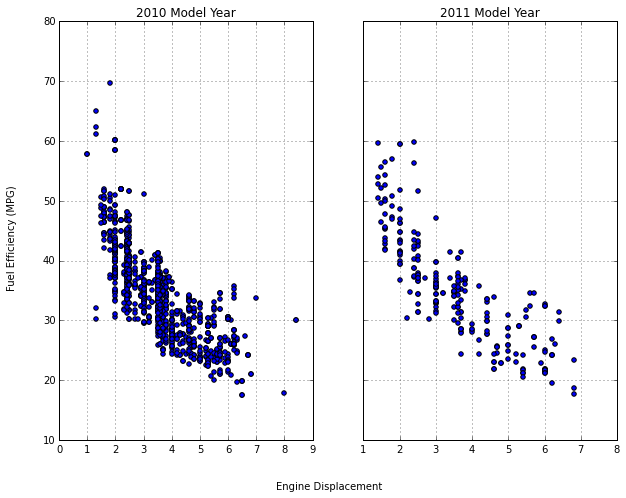Because of the nature of this problem, i.e. predict the MPG for a new car line, we take the 2010 data as training set and the 2011 data as test set.

In :
# Define the evaluation metric: root mean squared error (RMSE)
from sklearn.metrics import mean_squared_error

def rmse(y_actual, y_predicted):
'''calculate Root Mean Squared Error'''
return np.sqrt(mean_squared_error(y_actual, y_predicted))


A good starting point is the simple linear model $$y = \beta_0 + \beta_1x,$$ where $y$ is the Fuel Efficiency (MPG) and $x$ is the Engine Displacement.

In :
# simple linear model
from sklearn.linear_model import LinearRegression

reg = LinearRegression()
reg.fit(cars10_feature, cars10_target)
print "Least square estimate: intercept = {0}, coefficient ={1}".format(reg.intercept_, reg.coef_)

Least square estimate: intercept = [ 50.56322991], coefficient =[-4.52092928]

In :
X = np.linspace(np.min(cars10_feature), np.max(cars10_feature))[:, np.newaxis]
y = reg.predict(X)
cars10_target_pred = reg.predict(cars10_feature)
y_range = np.linspace(np.min(cars10_target), np.max(cars10_target))[:, np.newaxis]

fig, (ax1, ax2) = plt.subplots(1, 2)

ax1.scatter(cars10_feature, cars10_target)
ax1.plot(X, y, 'r')
ax1.set_title('2010 Model Year')
ax1.set_xlabel('Engine Displacement')
ax1.set_ylabel('Fuel Efficiency (MPG)')

ax2.scatter(cars10_target, cars10_target_pred)
ax2.plot(y_range, y_range, 'r--')
ax2.set_xlabel('Observed')
ax2.set_ylabel('Predicted')

Out:
<matplotlib.text.Text at 0x110f81190>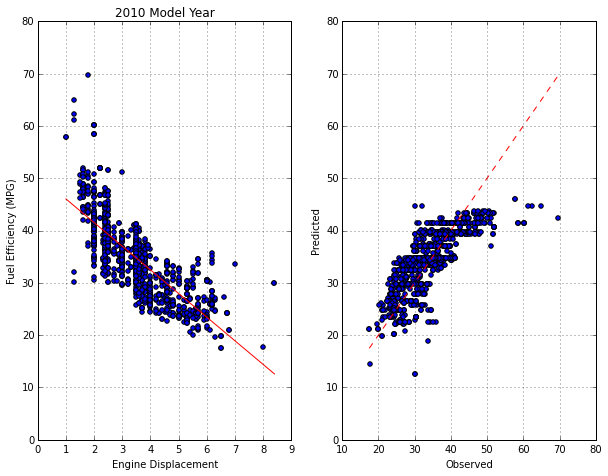The left-hand panel shows the training set data with a linear model fit defined by the estimated slope and intercept. The right-hand panel plots the observed and predicted MPG. These plots demonstrate that this model misses some of the patterns in the data, such as under-predicting fuel efficiency when the displacement is less than 2L or above 6L

In :
# calculate root mean square error (RMSE)
from sklearn.cross_validation import cross_val_score

scores = np.sqrt(np.abs(cross_val_score(reg, cars10_feature, cars10_target, cv=10, scoring='mean_squared_error')))
print "RMSE: {0}".format(np.mean(scores))

RMSE: 4.7277409602


Notice that simply re-predict the training set data is like to result in overly optimistic estimation of RMSE. An alternative approach for quantifying how well the model operates is to use resampling techniques, e.g. 10-fold cross-validation. We will cover that in Chapter 4.

Looking at the previous figure, it is conceivable that the problem might be solved by introducing some non-linearity in the model. The most basic approach is to supplement the simple linear model with additional complexity, e.g. $$y = \beta_0 + \beta_1 x + \beta_2 x^2,$$ which is referred to as quadratic model.

In :
# quadratic model
from sklearn.preprocessing import PolynomialFeatures
from sklearn.pipeline import make_pipeline

scores = np.sqrt(np.abs(cross_val_score(quad, cars10_feature, cars10_target, cv=10, scoring='mean_squared_error')))
print "RMSE: {0}".format(np.mean(scores))

RMSE: 4.34528462825


Reduce in the RMSE may suggest that this model is a better fit to the data.

In :
X = np.linspace(np.min(cars10_feature), np.max(cars10_feature))[:, np.newaxis]
y_range = np.linspace(np.min(cars10_target), np.max(cars10_target))[:, np.newaxis]

fig, (ax1, ax2) = plt.subplots(1, 2)

ax1.scatter(cars10_feature, cars10_target)
ax1.plot(X, y, 'r')
ax1.set_title('2010 Model Year')
ax1.set_xlabel('Engine Displacement')
ax1.set_ylabel('Fuel Efficiency (MPG)')

ax2.scatter(cars10_target, cars10_target_pred)
ax2.plot(y_range, y_range, 'r--')
ax2.set_xlabel('Observed')
ax2.set_ylabel('Predicted')

Out:
<matplotlib.text.Text at 0x112067c90>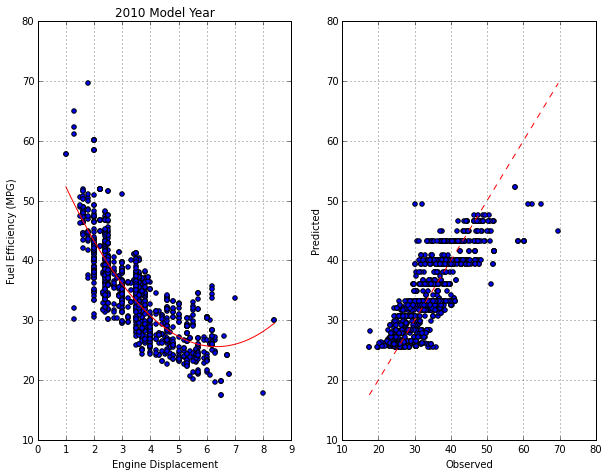One issue with quadratic models is that they can perform poorly on the extremes of the predictor. From the above figure, one might notice that predicting new vehicles with large displacement values may produce inaccurate results.

There are other approaches for creating sophisticated relationships between the predictors and outcome. One particular technique is the multivariate adaptive regression spline (MARS) model (Friedman (1991)). When used with a single predictor, MARS can fit separate linear regression lines for different ranges of engine displacement. This model, like many machine learning algorithms, has a tuning parameter which cannot be directly estimated from the data. While the MARS model has internal algorithms for making this dtermination, the user can try different values and use resampling to determin the appropriate value. Once the value is found, a final MARS model would be fit using all the training set data and used for prediction.

A Python module py-earth on Github implemented the MARS and is likely to be merged into sklearn in the near future (see this issue).

In :
# MARS
from pyearth import Earth

mars = Earth()
mars.fit(cars10_feature, cars10_target)
scores = np.sqrt(np.abs(cross_val_score(mars, cars10_feature, cars10_target, cv=10, scoring='mean_squared_error')))
print "RMSE: {0}".format(np.mean(scores))

RMSE: 4.38261607106


RMSE of MARS is similar to that of quadratic regression.

In :
X = np.linspace(np.min(cars10_feature), np.max(cars10_feature))[:, np.newaxis]
y = mars.predict(X)
cars10_target_pred = mars.predict(cars10_feature)
y_range = np.linspace(np.min(cars10_target), np.max(cars10_target))[:, np.newaxis]

fig, (ax1, ax2) = plt.subplots(1, 2)

ax1.scatter(cars10_feature, cars10_target)
ax1.plot(X, y, 'r')
ax1.set_title('2010 Model Year')
ax1.set_xlabel('Engine Displacement')
ax1.set_ylabel('Fuel Efficiency (MPG)')

ax2.scatter(cars10_target, cars10_target_pred)
ax2.plot(y_range, y_range, 'r--')
ax2.set_xlabel('Observed')
ax2.set_ylabel('Predicted')

Out:
<matplotlib.text.Text at 0x1120e41d0>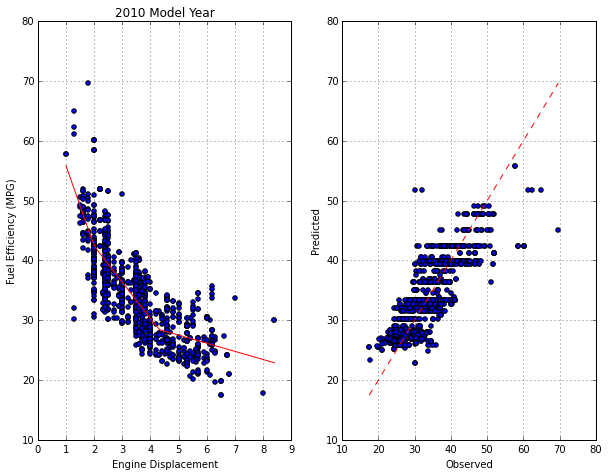Both quadratic model and MARS are evaluated on the test set.

In :
X = np.linspace(np.min(cars11_feature), np.max(cars11_feature))[:, np.newaxis]

fig, (ax1, ax2) = plt.subplots(1, 2)

ax1.scatter(cars11_feature, cars11_target)
ax1.set_xlabel('Engine Displacement')
ax1.set_ylabel('Fuel Efficiency (MPG)')

ax2.scatter(cars11_feature, cars11_target)
ax2.plot(X, mars.predict(X), 'r')
ax2.set_xlabel('Engine Displacement')
ax2.set_ylabel('Fuel Efficiency (MPG)')
ax2.set_title('MARS')

Out:
<matplotlib.text.Text at 0x1125ca450>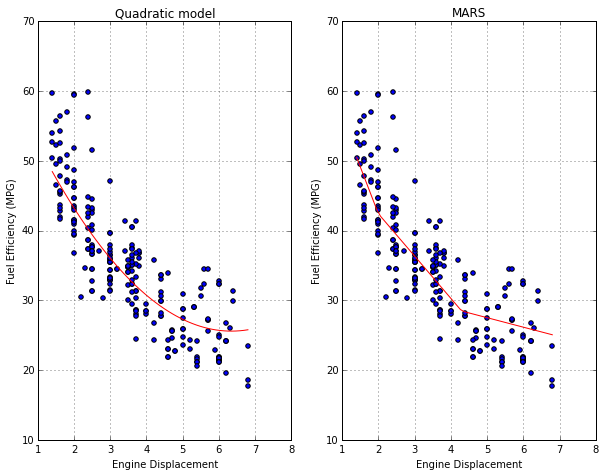In :
# RMSE
mars_scores = np.sqrt(np.abs(cross_val_score(mars, cars11_feature, cars11_target, cv=10, scoring='mean_squared_error')))


Quadratic model RMSE: 4.79365661288 and MARS RMSE: 4.83734524679


The first thing to notice is that both scores are very similar, which indicates that either model is appropriate for this task. Also, both scores are lower than their previous values (fitted to 2010 data) as we would expect.

## 2.2 Themes¶

There are several aspects of the model building process that are worth discussing further.

### Data Splitting¶

• How we allocate data to certain tasks, e.g. model building, evaluating performance?
• extrapolation: order matters
• interpolation: a simple random sample of the data
• How much data should be allocated to the training and test sets?
• small data sets: resampling techniques, i.e. no test set
• large data sets

### Predictor Data¶

Feature selection: the process of determining the minimum set of relevant predictors needed by the model.

### Estimating Performance¶

• Quantitative assessments of statistics (using resampling techniques)
• Visualization

### Evaluating Several Models¶

"No Free Lunch" Theorem - Try a wide variety of techniques then determine which model to focus on.

### Model Selection¶

• between models
• within the same model

Rely on cross-validation and the test set to produce quantitative assessments of the models to make the choice.

## 2.3 Summary¶

To get a reliable, trustworthy model for predicting new samples, we must first understand the data and the objective of the modeling.